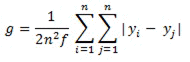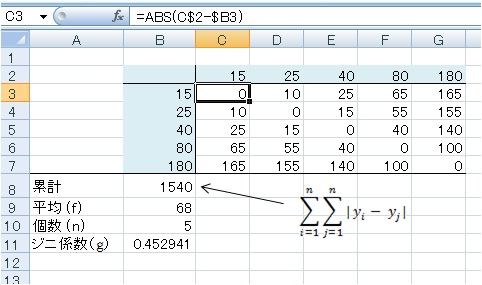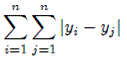Japanese

## How to get Gini's coefficient using Excel

Gini's coefficient is easily calculated with the use of Excel Application. This coefficient measures the degree of inequality in income of community. This value ranges between 0 to 1, where extreme zero value means an ideal equlity.

Gini's coefficient is defined as, where éÖéP, éÖ2, ücüc éÖn are distribution of individual income of n samples,
and f is an average value of them.
Therefore f is given as f = ( éÖéP + éÖ2 + ücüc + éÖn) / nThe values éÖéP, éÖ2, ücéÖn are filled in painted cells as demonstrated in the figure above.

C3 cell is typed as üw =abs(c\$2-\$b3) üx , and all cells in the table are copied entirely.

(note) Excel's ABS-function gives Absolute value, i.e. | 15 - 40 | equals to 25.

B8 cell is typed asüw =sum(c3:g7) üx , indicating thevalue in the Gini's definition.

B9 is an average value of n samples i.e. üw =average(b3:b7) üx and B10 consists of üw =count(b3:b7) üx, meaning the count of values corresponding the number of sample (n).

Gini's coefficient, B11 cell is calculated as üw =b8/(2*b10*b10*b9) üx.

Description of B11 is also available as üw =b8/(2*b10^2*b9) üx. üw ^2 üx covers a value of number square, and üw ^3 üx covers that of cubic.

You can get a similar result if you copy the subsequent table and paste on your Excel Sheet. The item "sample" must be set in A1 cell.

 sample 15 25 40 80 180 15 =ABS(C\$2-\$B3) =ABS(D\$2-\$B3) =ABS(E\$2-\$B3) =ABS(F\$2-\$B3) =ABS(G\$2-\$B3) 25 =ABS(C\$2-\$B4) =ABS(D\$2-\$B4) =ABS(E\$2-\$B4) =ABS(F\$2-\$B4) =ABS(G\$2-\$B4) 40 =ABS(C\$2-\$B5) =ABS(D\$2-\$B5) =ABS(E\$2-\$B5) =ABS(F\$2-\$B5) =ABS(G\$2-\$B5) 80 =ABS(C\$2-\$B6) =ABS(D\$2-\$B6) =ABS(E\$2-\$B6) =ABS(F\$2-\$B6) =ABS(G\$2-\$B6) 180 =ABS(C\$2-\$B7) =ABS(D\$2-\$B7) =ABS(E\$2-\$B7) =ABS(F\$2-\$B7) =ABS(G\$2-\$B7) total amount =SUM(C3:G7) average (f) =AVERAGE(B3:B7) samples (n) =COUNT(B3:B7) Gini's coeff.üiéćüj =B8/(2*B10*B10*B9)